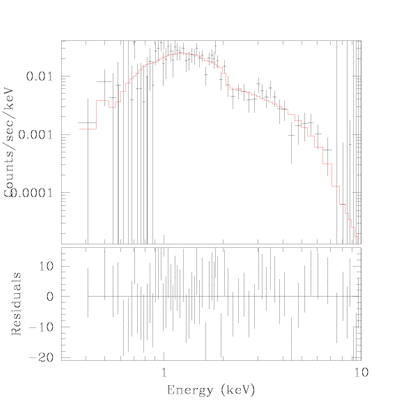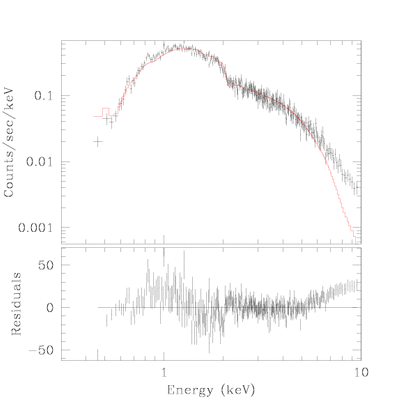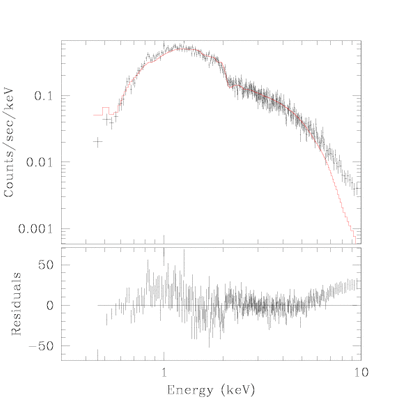True Color Image Gallery: Galactic SNR | Magellanic Cloud SNR

# Fit Detail for SNRJ 0540.1-6919

nH was derived by fitting the trail of central source which isbelieved not to be piled-up.

## trail:

source=(xswabs * powlaw1d)
reduce chi^2 =
nh = 0.4591 10^22/cm^2
PARAMETERSDataSet 1: 67 data points -- 64 degrees of freedom.
• Statistic value = 53.6161
• Probability [Q-value] = 0.819365
• Reduced statistic = 0.837752
--end--

## central source:

• while spectrum from the central source is stronly affected by pile-up, derived nH is similar.
source=(xswabs * powlaw1d)
reduce chi^2 =
nh = 0.4737 10^22/cm^2
PARAMETERSDataSet 2: 315 data points -- 312 degrees of freedom.
• Statistic value = 621.508
• Probability [Q-value] = 9.61303e-23
• Reduced statistic = 1.99201
--end--

## central source(2):

• spectrum was refitted with nH fixed at the value derived from the trail of central source.
source=(xswabs * powlaw1d)
reduce chi^2 =
nh = 0.4591 10^22/cm^2
PARAMETERSDataSet 2: 315 data points -- 313 degrees of freedom.
• Statistic value = 624.558
• Probability [Q-value] = 6.31621e-23
• Reduced statistic = 1.99539
--end--

## shell:

• shell was fitted by 3 thermal plasma model with nH fixed.
source=(xswabs * ((xsraymond + xsraymond) + xsraymond))
reduce chi^2 =
nh = 0.4591 10^22/cm^2
PARAMETERSDataSet 3: 157 data points -- 151 degrees of freedom.
• Statistic value = 405.329
• Probability [Q-value] = 3.79852e-25
• Reduced statistic = 2.6843
--end--

## total:

• sum of the spectral models from central source and shell.
• no futher fitting was done.
• Hwang et al.(2001) derived nH=0.2~0.5 for various part of the shell(dimension of the nH in their table seems to be a typo(10^22 instead of 10^21)).
source=(xswabs * (((powlaw1d + xsraymond) + xsraymond) + xsraymond))
reduce chi^2 =
nh = 0.4591 10^22/cm^2
PARAMETERSDataSet 5: 353 data points -- 345 degrees of freedom.
• Statistic value = 876.573
• Probability [Q-value] = 0
• Reduced statistic = 2.54079
--end--

Back to SNRJ 0540.1-6919 IndexChandra Supernova Remnant CatalogMain Page | Galactic SNR | Magellanic Cloud SNRTrue Color Image Gallery: Galactic SNR | Magellanic Cloud SNR